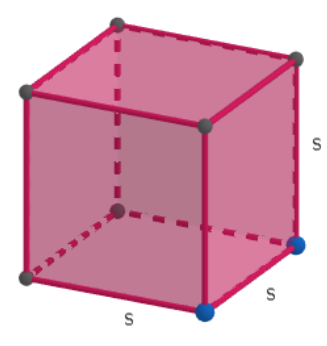QuestionAnswers

# If eight cubes are stacked to form a big cube, then find the percentage decrease in the total surface area.(a) 30 %(b) 40 %(c) 50 %(d) 60 %

Hint: To solve this question, we will assume some variables for the length of the smaller cubes and find the surface area of the smaller cubes, given as the sum of areas of all the sides, that is six times the square of the length of the side. The collective surface area will be 8 times the surface area of each cube. We will also find the volume of the smaller cube given as the cube of the length of the side. Eight times the volume of a smaller cube will be the volume of a bigger cube. Thus, we will find the length of the bigger cube. Once we get the length, we can get the surface area. The percentage decrease in the surface area will be given by the relation $pd=\dfrac{{{a}_{1}}-{{a}_{2}}}{{{a}_{1}}}\times 100\%$ , where ‘pd’ is percentage decrease, ${{a}_{1}}$ is cumulative surface area of 8 smaller squares and ${{a}_{2}}$ is the surface area of the bigger square.

Complete step-by-step solution:
Let the length of the smaller cube be s.Let A be the surface area of the smaller cube. It is given as 6 times the square of the length of the side.
$\Rightarrow A=6{{s}^{2}}$
Let ${{a}_{1}}$ be the cumulative surface area of 8 cubes. It will be 8 times surface area of single cube.
\begin{align} & \Rightarrow {{a}_{1}}=8\times 6{{s}^{2}} \\ & \Rightarrow {{a}_{1}}=48{{s}^{2}}......\left( 1 \right) \\ \end{align}
Now, the volume of a cube is given as the cube of the length of the side.
Let v be the volume of one smaller cube.
$\Rightarrow v={{s}^{3}}$
Let ${{v}_{1}}$ be the cumulative volume of 8 smaller cubes. It will be 8 times the volume of one smaller cube.
$\Rightarrow {{v}_{1}}=8{{s}^{3}}$
Now, we know that the volume of the cubes will be conserved. Hence, cumulative volume of 8 smaller cubes will be equal to the volume of one bigger cube.
If V is the volume of the bigger cube, then $V={{v}_{1}}$.
$\Rightarrow V=8{{s}^{3}}$
Let a be the length of the side of the bigger cube.
The volume of the bigger cube is given as the cube of the length of the side of the bigger cube.
\begin{align} & \Rightarrow V={{a}^{3}} \\ & \Rightarrow {{a}^{3}}=8{{s}^{3}} \\ & \Rightarrow a=2s \\ \end{align}
Thus, the length of the side of the bigger cube is 2s.
Let ${{a}_{2}}$ be the surface area of the bigger cube. It will be six times the square of the length of the side.
\begin{align} & \Rightarrow {{a}_{2}}=6{{\left( 2s \right)}^{2}} \\ & \Rightarrow {{a}_{2}}=6\times 4{{s}^{2}} \\ & \Rightarrow {{a}_{2}}=24{{s}^{2}}......\left( 2 \right) \\ \end{align}
The percentage decrease in the surface area is given by the relation $pd=\dfrac{{{a}_{1}}-{{a}_{2}}}{{{a}_{1}}}\times 100%$ , where pd is percentage decrease, ${{a}_{1}}$ is cumulative surface area of 8 smaller squares and ${{a}_{2}}$ is the surface area of the bigger square.
We will substitute ${{a}_{1}}=48{{s}^{2}}$ and ${{a}_{2}}=24{{s}^{2}}$ in $pd=\dfrac{{{a}_{1}}-{{a}_{2}}}{{{a}_{1}}}\times 100\%$
\begin{align} & \Rightarrow pd=\dfrac{48{{s}^{2}}-24{{s}^{2}}}{48{{s}^{2}}}\times 100\% \\ & \Rightarrow pd=\dfrac{1}{2}\times 100\% \\ & \Rightarrow pd=50\% \\ \end{align}
Hence, option (c) is the correct option.

Note: We need to follow the conservation of mass and volume. The conservation of mass and volume states that when we stack smaller pieces to form a bigger piece, the mass and thus the volume remains the same if there is no loss of material.